# NCERT Exemplar Class 9 Science Solutions for Chapter 12 - Sound

## NCERT Exemplar Solutions Class 9 Science Chapter 12 – Free PDF Download

NCERT Exemplar Solutions for Class 9 Science Chapter 12 Sound are designed in such a way that students can answer different types of questions asked in the annual examination without any difficulty. Important concepts related to sound are sound propagation, the production of sound, the range of hearing and the application of ultrasound. These solutions provide answers to the questions given in the NCERT Exemplar book. NCERT Exemplar Solutions for Class 9 Science contain different types of questions like numerical questions, MCQs, short answer questions and long answer questions.

### Download the PDF of NCERT Exemplar Solutions for Class 9 Science Chapter 12 – Sound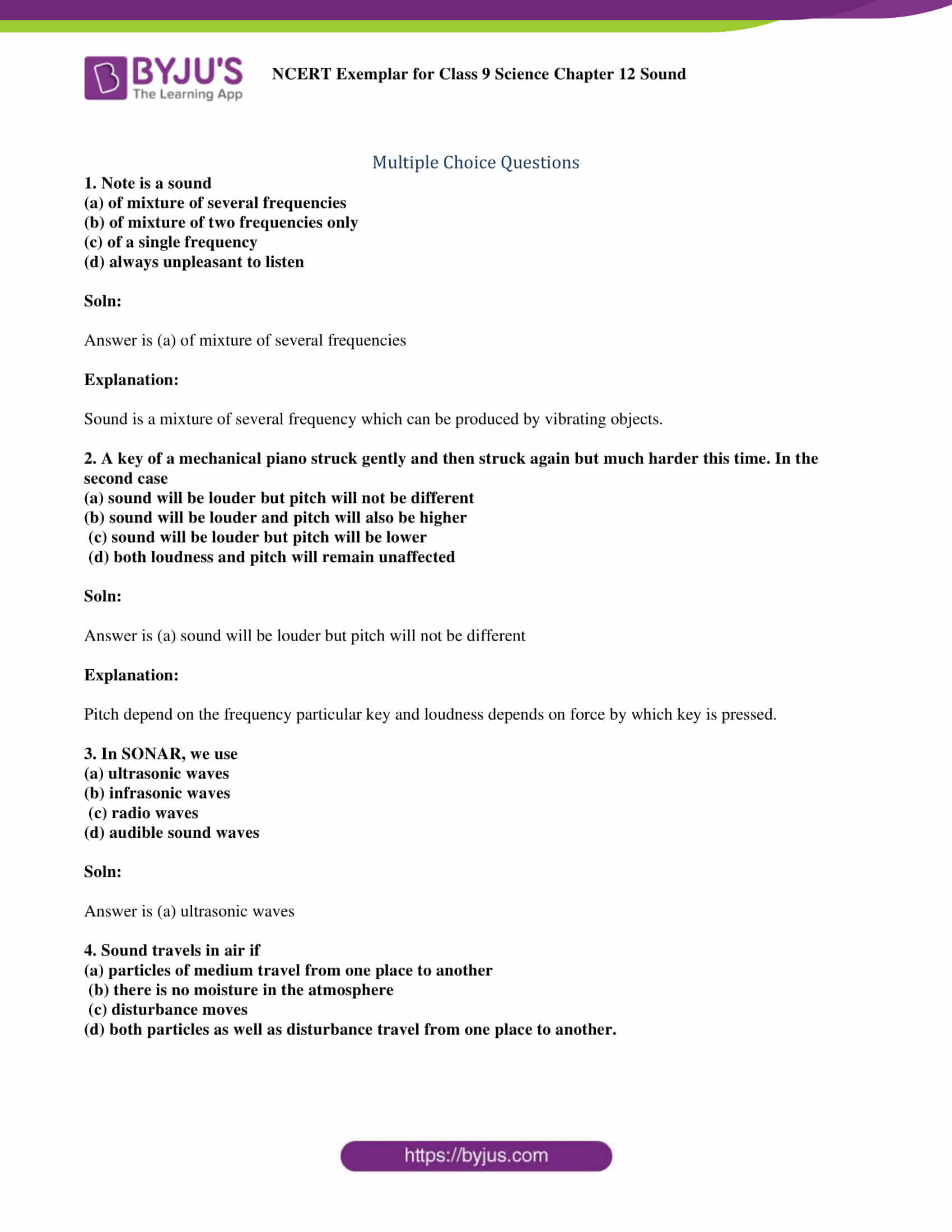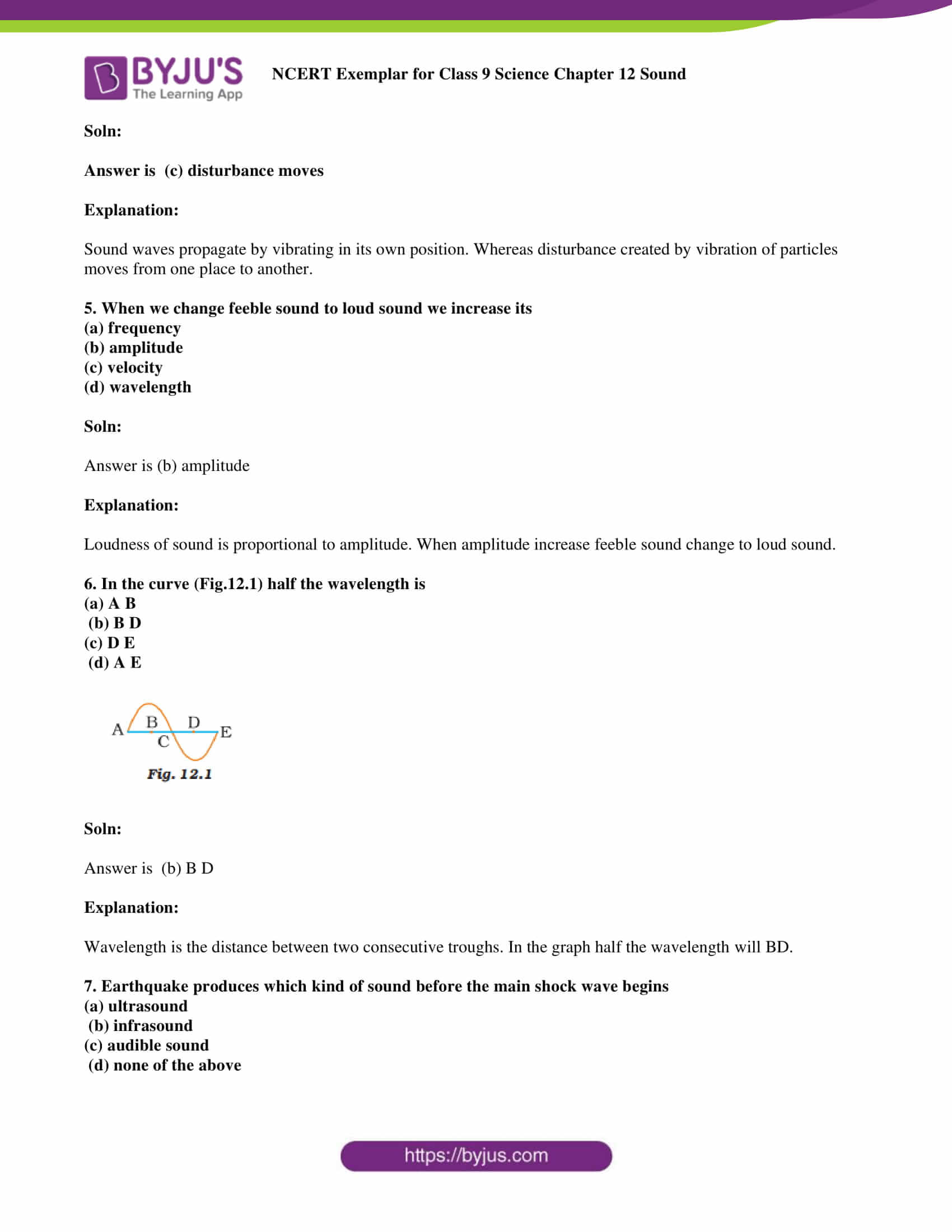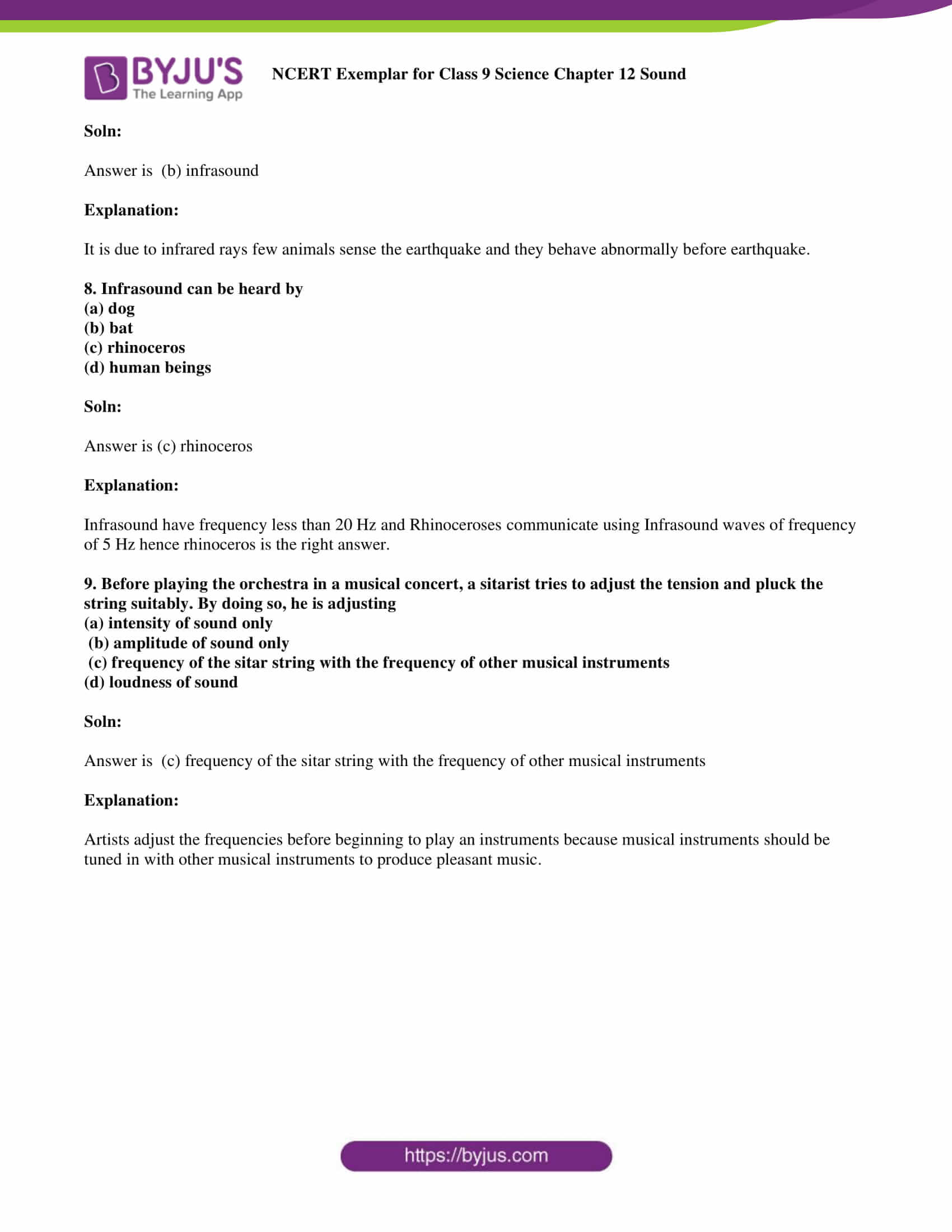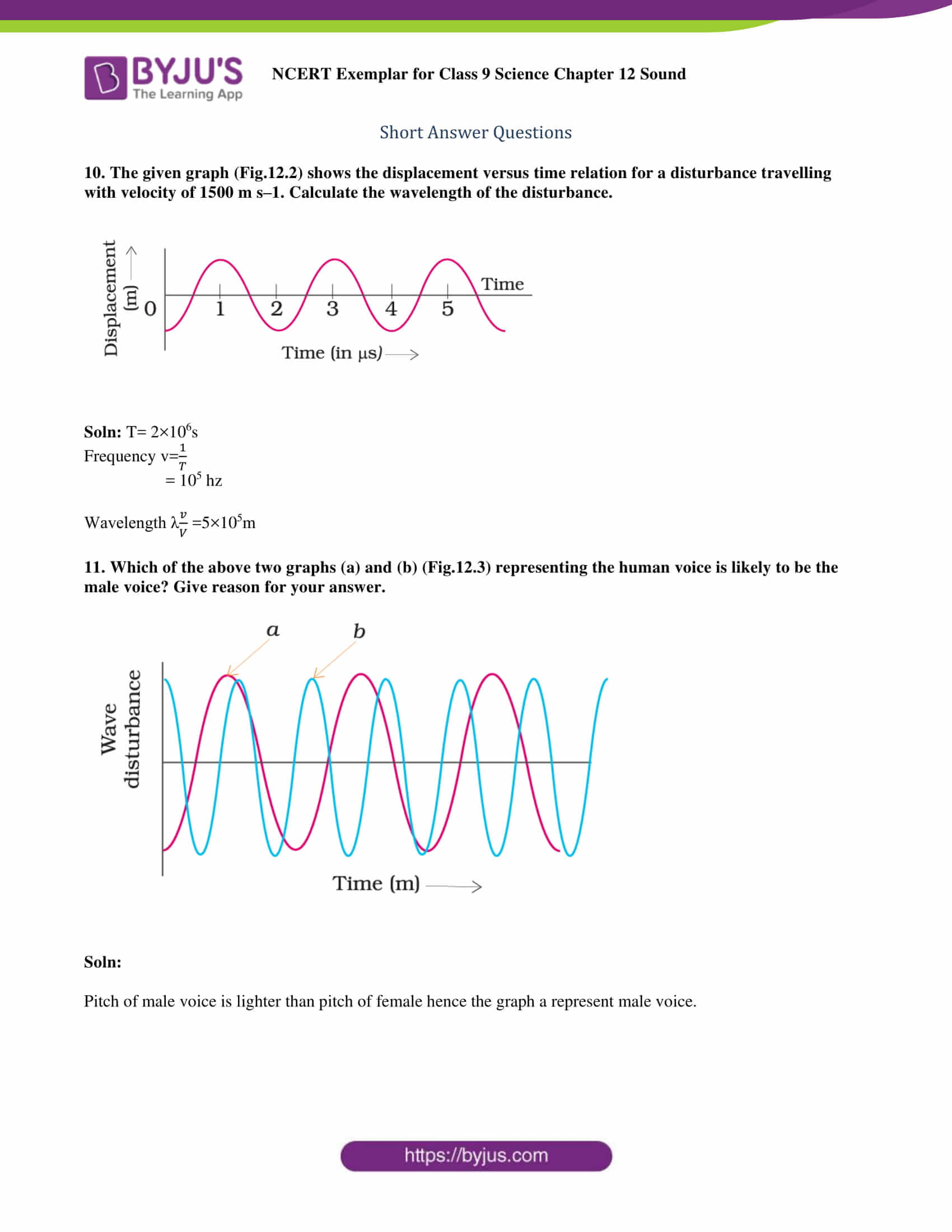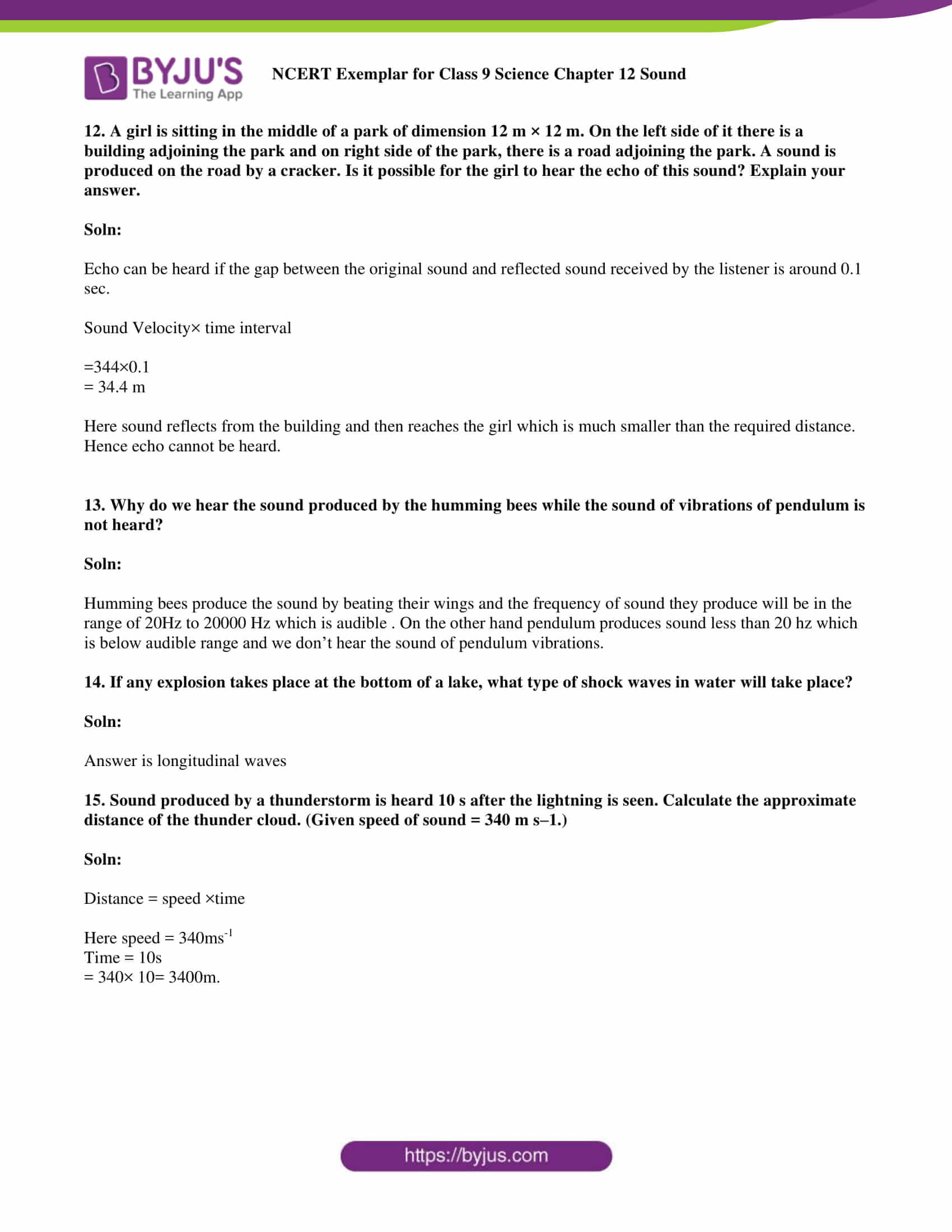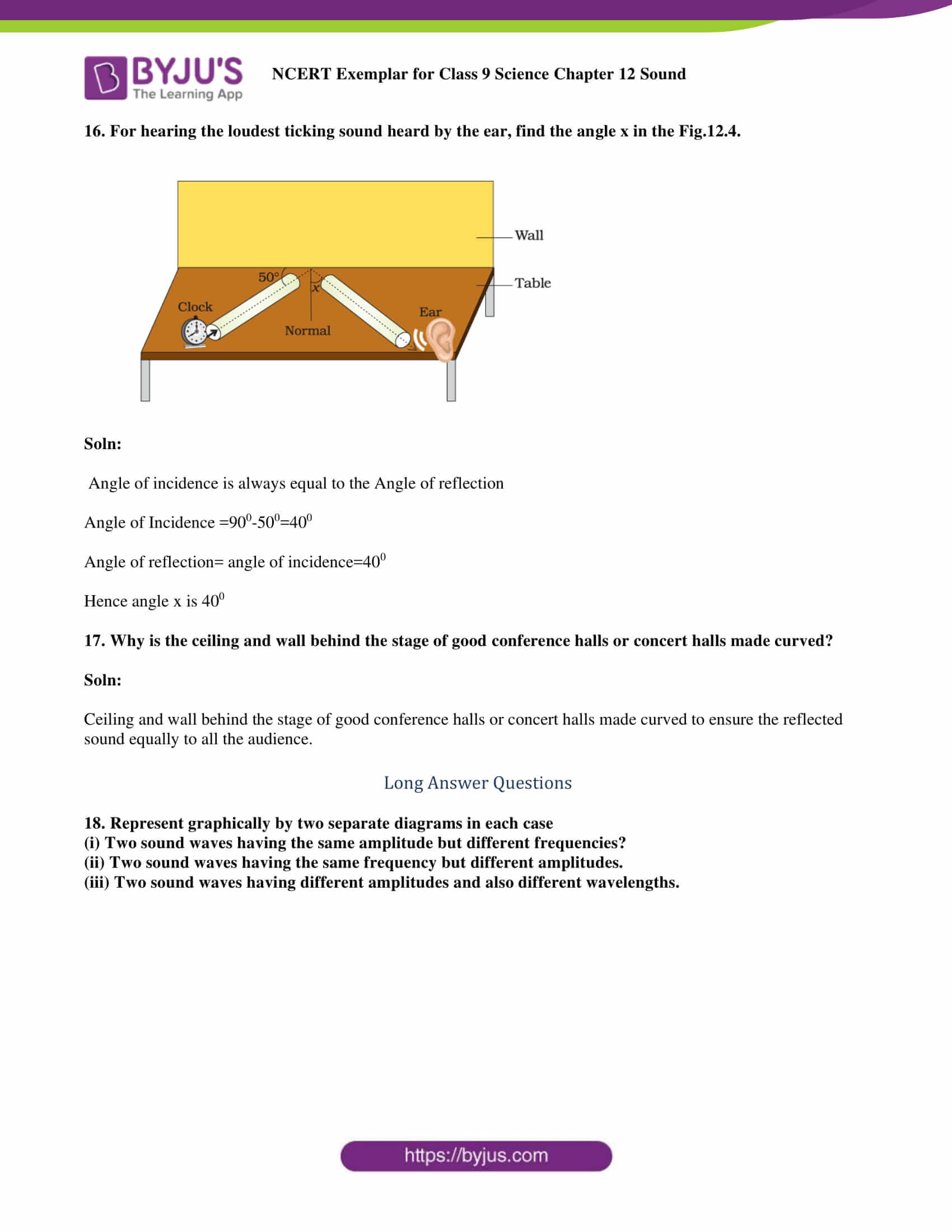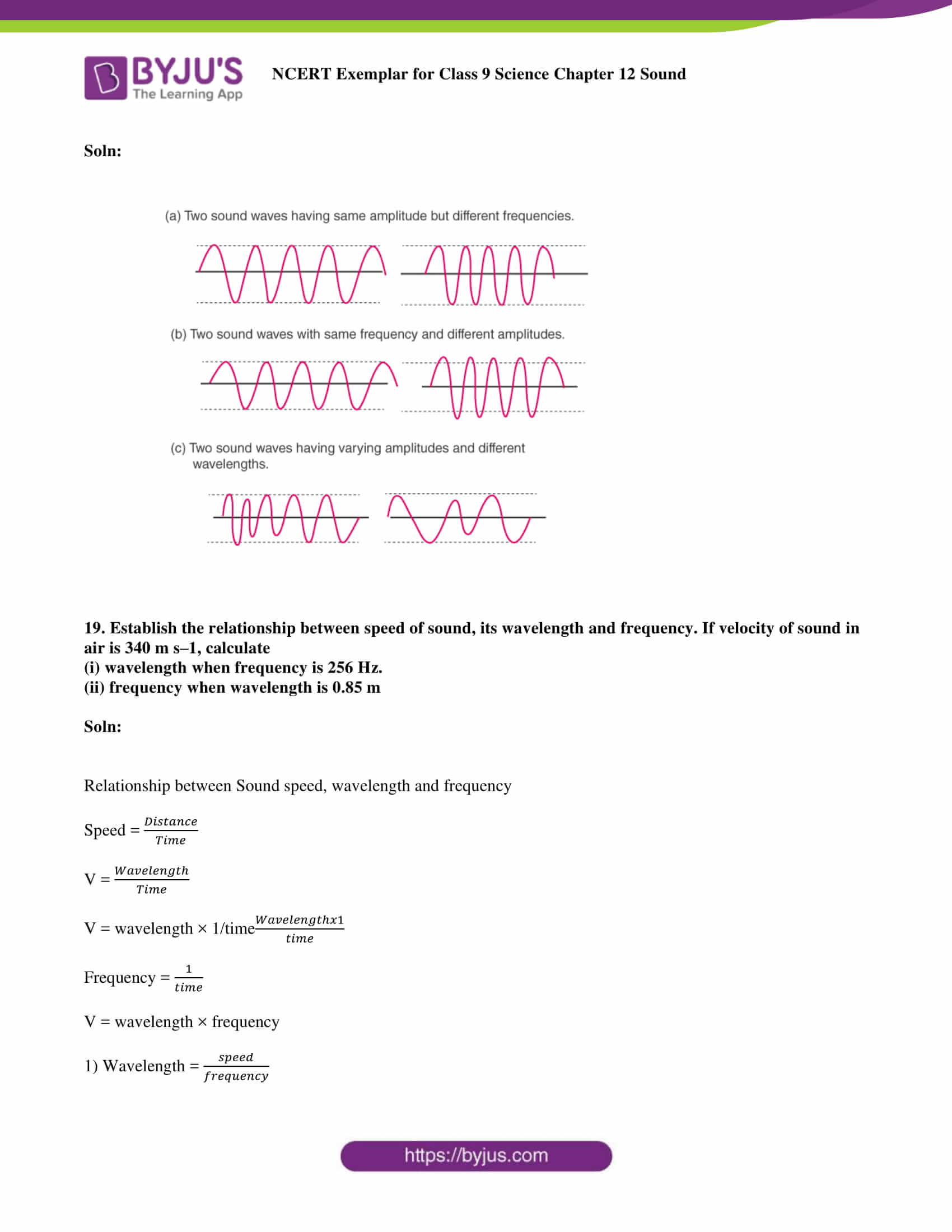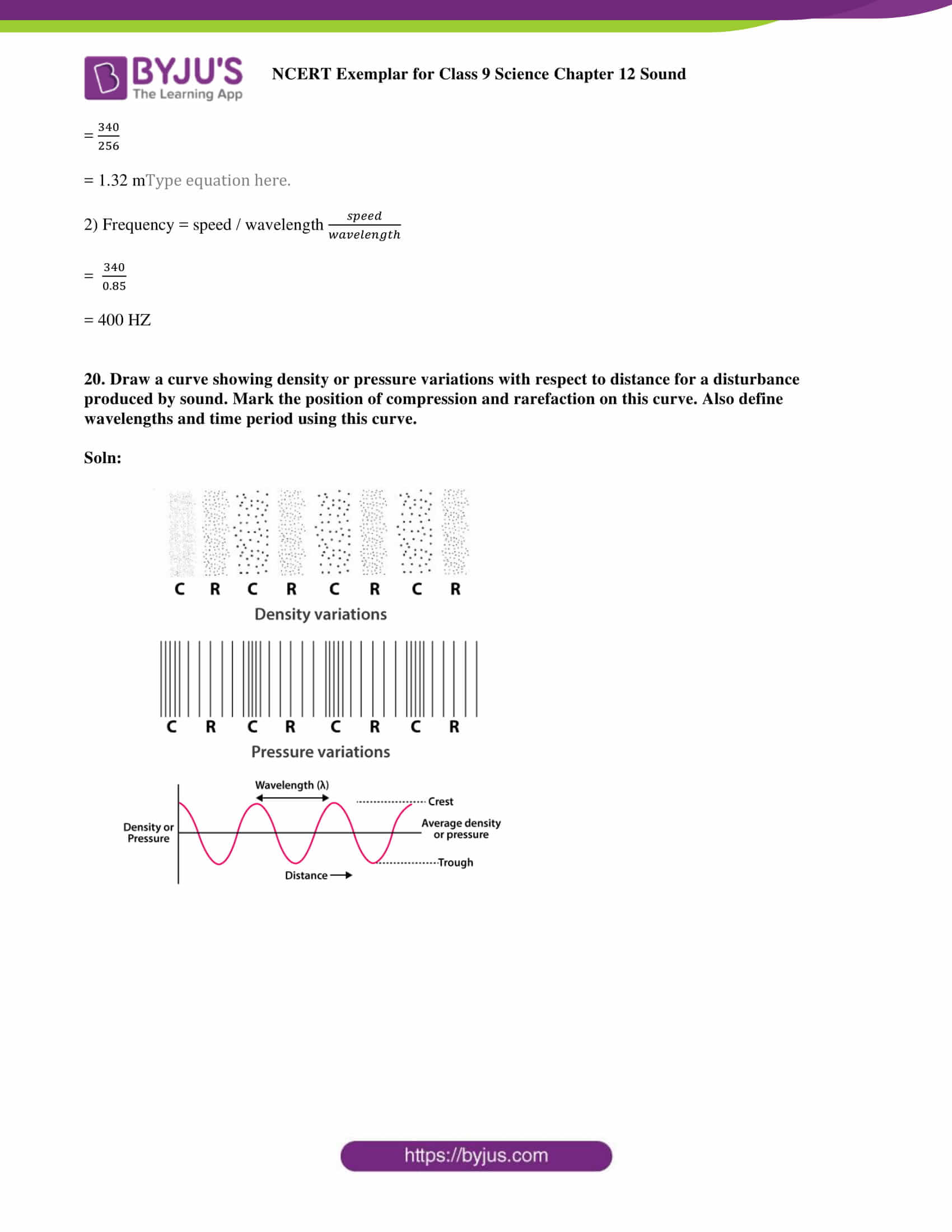### Multiple Choice Questions

1. Note is a sound

(a) of mixture of several frequencies

(b) of mixture of two frequencies only

(c) of a single frequency

(d) always unpleasant to listen

Soln:

Answer is (a) of mixture of several frequencies

Explanation:

Sound is a mixture of several frequencies which can be produced by vibrating objects.

2. A key of a mechanical piano struck gently and then struck again but much harder this time. In the second case

(a) sound will be louder, but the pitch will not be different

(b) sound will be louder, and the pitch will also be higher

(c) sound will be louder, but the pitch will be lower

(d) both loudness and pitch will remain unaffected

Soln:

Answer is (a) sound will be louder, but the pitch will not be different

Explanation:

Pitch depends on the frequency particular key, and loudness depends on the force by which the key is pressed.

3. In SONAR, we use

(a) ultrasonic waves

(b) infrasonic waves

(d) audible sound waves

Soln:

4. Sound travels in air if

(a) particles of medium travel from one place to another

(b) there is no moisture in the atmosphere

(c) disturbance moves

(d) both particles as well as disturbance, travel from one place to another.

Soln:

Explanation:

Sound waves propagate by vibrating in their own position. Whereas disturbance created by the vibration of particles moves from one place to another.

5. When we change feeble sound to loud sound, we increase its

(a) frequency

(b) amplitude

(c) velocity

(d) wavelength

Soln:

Explanation:

The Loudness of sound is proportional to amplitude. When amplitude increases, feeble sound changes to loud sound.

6. In the curve (Fig.12.1), half the wavelength is

(a) A B

(b) B D

(c) D E

(d) A E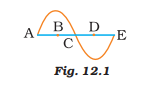Soln:

Explanation:

Wavelength is the distance between two consecutive troughs. In the graph half, the wavelength will be BD.

7. Earthquake produces which kind of sound before the main shock wave begins

(a) ultrasound

(b) infrasound

(c) audible sound

(d) none of the above

Soln:

Explanation:

It is due to infrared rays, few animals sense the earthquake, and they behave abnormally before the earthquake.

8. Infrasound can be heard by

(a) dog

(b) bat

(c) rhinoceros

(d) human beings

Soln:

Explanation:

Infrasound has a frequency less than 20 Hz, and Rhinoceroses communicate using Infrasound waves of the frequency of 5 Hz hence rhinoceros is the right answer.

9. Before playing the orchestra in a musical concert, a sitarist tries to adjust the tension and pluck the string suitably. By doing so, he is adjusting

(a) intensity of sound only

(b) amplitude of sound only

(c) frequency of the sitar string with the frequency of other musical instruments

(d) loudness of sound

Soln:

Answer is (c) frequency of the sitar string with the frequency of other musical instruments

Explanation:

Artists adjust the frequencies before beginning to play instruments because musical instruments should be tuned in with other musical instruments to produce pleasant music.

10. The given graph (Fig.12.2) shows the displacement versus time relation for a disturbance travelling with a velocity of 1500 m s–1. Calculate the wavelength of the disturbance.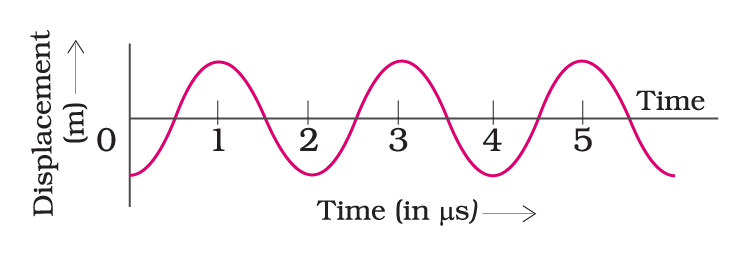Soln: T= 2×106s

Frequency v= 1/T

= 105 hz

Wavelength λ v/V=5×105m

11. Which of the above two graphs (a) and (b) (Fig.12.3) representing the human voice is likely to be the male voice? Give the reason for your answer.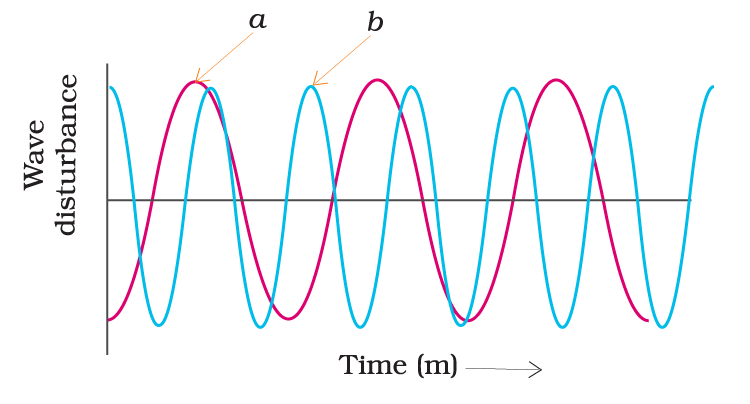Soln:

The pitch of a male voice is lighter than the pitch of a female; hence the graph represents the male voice.

12. A girl is sitting in the middle of a park of dimension 12 m × 12 m. On the left side of it, there is a building adjoining the park, and on the right side of the park, there is a road adjoining the park. A sound is produced on the road by a cracker. Is it possible for the girl to hear the echo of this sound? Explain your answer.

Soln:

Echo can be heard if the gap between the original sound and the reflected sound received by the listener is around 0.1 sec.

Sound Velocity × time interval

= 344×0.1

= 34.4 m

Here, sound reflects from the building and then reaches the girl, who is much smaller than the required distance. Hence echo cannot be heard.

13. Why do we hear the sound produced by the humming bees while the sound of vibrations of a pendulum is not heard?

Soln:

Humming bees produce the sound by beating their wings, and the frequency of the sound they produce will be in the range of 20 Hz to 20000 Hz, which is audible. On the other hand, the pendulum produces sound less than 20 Hz, which is below the audible range, and we don’t hear the sound of pendulum vibrations.

14. If any explosion takes place at the bottom of a lake, what type of shock waves in the water will take place?

Soln:

15. The sound produced by a thunderstorm is heard 10 s after the lightning is seen. Calculate the approximate distance of the thundercloud. (Given the speed of sound = 340 m s–1.)

Soln:

Distance = speed ×time

Here speed = 340ms-1

Time = 10s

= 340× 10 = 3400m.

16. For hearing the loudest ticking sound heard by the ear, find the angle x in Fig.12.4.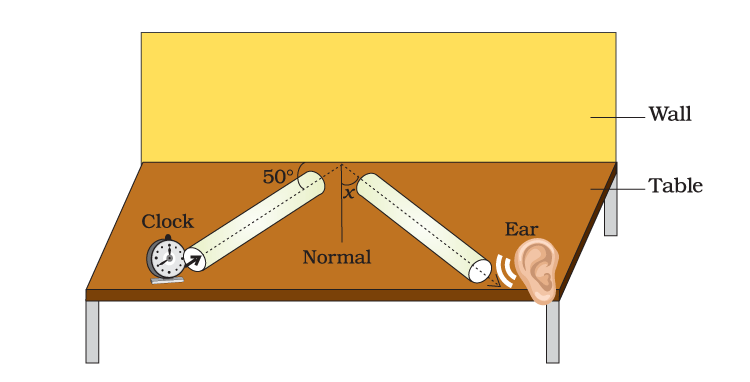Soln:

The angle of incidence is always equal to the Angle of reflection

Angle of Incidence =900-500=400

Angle of reflection= angle of incidence=400

Hence angle x is 400

17. Why are the ceiling and wall behind the stage of good conference halls or concert halls made curved?

Soln:

The ceiling and wall behind the stage of good conference halls or concert halls made curved to ensure the reflected sound reaches equally to all the audience.

18. Represent graphically by two separate diagrams in each case

(i) Two sound waves having the same amplitude but different frequencies?

(ii) Two sound waves having the same frequency but different amplitudes.

(iii) Two sound waves having different amplitudes and also different wavelengths.

Soln: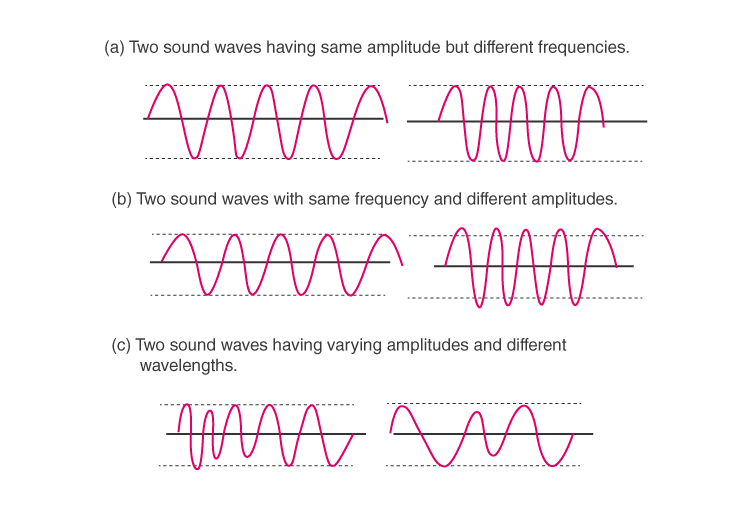19. Establish the relationship between the speed of sound, its wavelength and frequency. If the velocity of sound in air is 340 m s–1, calculate

(i) wavelength when the frequency is 256 Hz.

(ii) frequency when the wavelength is 0.85 m

Soln:

Relationship between Sound speed, wavelength and frequency

Speed = distance/time

V = wavelength/time

V = wavelength × 1/time x wavelength x 1 /time

Frequency = 1/time

V = wavelength × frequency

1) Wavelength = speed/frequency

=340/256

= 1.32 m

2) Frequency = speed / wavelength

=340/0.85

= 400 HZ

20. Draw a curve showing density or pressure variations with respect to distance for a disturbance produced by sound. Mark the position of compression and rarefaction on this curve. Also, define wavelengths and time periods using this curve.

Soln: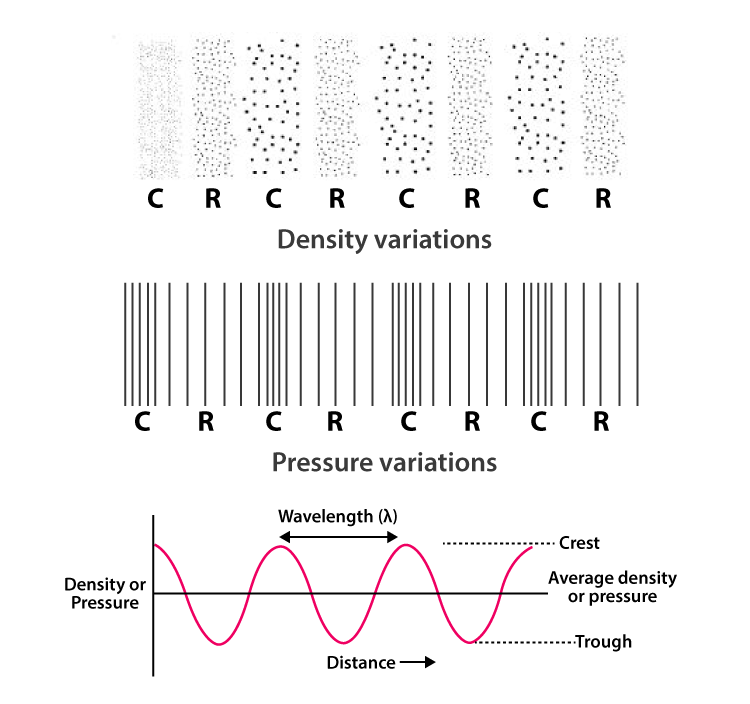### Topics Covered in Exemplar Solutions for Class 9 Science Chapter 12 Sound

• Production of sounds
• Propagation of sound
• Sound waves are longitudinal waves
• Characteristics of the sound wave
• The pace of sound in different media
• Reflection of sound
• Echo
• Reverberation
• Range of hearing
• Applications of ultrasound
• Sonar
• Structure of the human ear

Physics is a tough subject for many students, and they often struggle to memorise the formulas and derivations. To help students memorise the concepts and formulas, BYJU’S brings you videos, animations, study materials, notes, NCERT Solutions, sample papers and previous years’ question papers. To get access to all the study resources provided by us, register with BYJU’S or download BYJU’S – The Learning App.

## Frequently Asked Questions on NCERT Exemplar Solutions for Class 9 Science Chapter 12

Q1

### List out the topics covered in Chapter 12 of NCERT Exemplar Solutions for Class 9 Science.

The topics covered in Chapter 12 of NCERT Exemplar Solutions for Class 9 Science are listed below:
1. Production of sounds
2. Propagation of sound
3. Sound waves are longitudinal waves
4. Characteristics of the sound wave
5. The pace of sound in different media
6. Reflection of sound
7. Echo
8. Reverberation
9. Range of hearing
10. Applications of ultrasound
11. Sonar
12. Structure of the human ear
Q2

### Why should the students download the NCERT Exemplar Solutions for Class 9 Science Chapter 12 PDF?

The NCERT Exemplar Solutions for Class 9 Science Chapter 12 PDF contain answers to all the questions present in the textbook, along with diagrams. Each and every question is answered by keeping in mind the understanding abilities of students. The solutions are created strictly adhering to the latest CBSE syllabus and exam pattern to help students to face the annual exams confidently. It also improves their time management skills which are important from the exam point of view.
Q3

### Explain the concept of reverberation present in Chapter 12 of NCERT Exemplar Solutions for Class 9 Science.

Reverberation is the phenomenon of the persistence of sound after it has been stopped as a result of multiple reflections from surfaces, such as furniture, people, air, etc., within a closed surface. These reflections build up with each reflection and decay gradually as they are absorbed by the surfaces of objects in the enclosed space. Reverberations do wonders when it comes to musical symphonies and orchestra halls, when the right amount of reverberation is present, the sound quality gets enhanced drastically. This is the reason why sound engineers are appointed during the construction of these halls.